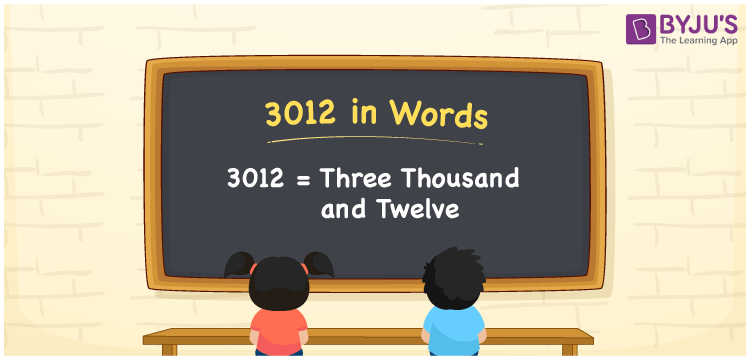# 3012 in words

3012 in words is written as Three Thousand and Twelve. 3012 represents the count or value. The article on Counting Numbers can give you an idea about count or counting. The number 3012 is used in expressions that relate to money, distance, length, year and others. Let us consider an example for 3012. ”The distance between point A to point B is Three Thousand and Twelve kilometers.”

 3012 in words Three Thousand and Twelve Three Thousand and Twelve in Numbers 3012

## 3012 in English Words## How to Write 3012 in Words?

We can convert 3012 to words using a place value chart. The number 3012 has 4 digits, so let’s make a chart that shows the place value up to 4 digits.

 Thousands Hundreds Tens Ones 3 0 1 2

Thus, we can write the expanded form as:

3 × Thousand + 0 × Hundred + 1 × Ten + 2 × One

= 3 × 1000 + 0 × 100 + 1 × 10 + 2 × 1

= 3012

= Three Thousand and Twelve.

3012 is the natural number that is succeeded by 3011 and preceded by 3013.

3012 in words – Three Thousand and Twelve.

Is 3012 an odd number? – No.

Is 3012 an even number? – Yes.

Is 3012 a perfect square number? – No.

Is 3012 a perfect cube number? – No.

Is 3012 a prime number? – No.

Is 3012 a composite number? – Yes.

## Solved Example

1. Write the number 3012 in expanded form

Solution: 3 × 1000 + 0 × 100 + 1 × 10 + 2 × 1

We can write 3012 = 3000 + 000 + 10 + 2

= 3 × 1000 + 0 × 100 + 1 × 10 + 2 × 1

## Frequently Asked Questions on 3012 in words

Q1

### How to write 3012 in words?

3012 in words is written as Three Thousand and Twelve.
Q2

### Is 3012 a perfect square number?

No. 3012 is not a perfect square number.
Q3

### Is 3012 a prime number?

No. 3012 is not a prime number.